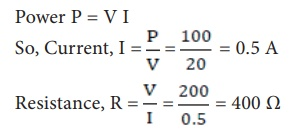Home | | Science 10th Std | Solved Problems

# Solved Problems

Science : Electricity: Solved Example Problems with Answers, Solution

Electricity - Science

Solved Problems

1. Two bulbs are having the ratings as 60 W, 220 V and 40 W, 220 V respectively. Which one has a greater resistance?

### Solution:

Electric power P = V2/ R

For the same value of V, R is inversely proportional to P.

Therefore, lesser the power, greater the resistance

Hence, the bulb with 40 W, 220 V rating has a greater resistance.

2. Calculate the current and the resistance of a 100 W, 200 V electric bulb in an electric circuit

### Solution:

Power P = 100 W and Voltage V = 200 V

Power P = V I3. In the circuit diagram given below, three resistors R1, R2 and R3 of 5 â„¦, 10 â„¦ and 20 â„¦ respectively are connected as shown. Calculate:A) Current through each resistor

B) Total current in the circuit

C) Total resistance in the circuit

### Solution:

A) Since the resistors are connected in parallel, the potential difference across each resistor is same (i.e. V=10V)

Therefore, the current through R1 is,B) Total current in the circuit, I = I1 + I2 + I3

= 2 + 1 + 0.5 = 3.5 A

4. Three resistors of 1 â„¦, 2 â„¦ and 4 â„¦ are connected in parallel in a circuit. If a 1 â„¦ resistor draws a current of 1 A, find the current through the other two resistors.

### Solution:

R1 = 1 â„¦, R2 = 2 â„¦, R3 = 4 â„¦ Current I1 = 1 A

The potential difference across the 1 â„¦ resistor = I1 R1 = 1 Ă— 1 = 1 V

Since, the resistors are connected in parallel in the circuit, the same potential difference will exist across the other resistors also.

So, the current in the 2 â„¦ resistor, V/R2= 1/2= 0.5 A

Similarly, the current in the 4 â„¦ resistor,

V/R2 = 1/4 = 0.25 A

Tags : Electricity | Science , 10th Science : Chapter 4 : Electricity
Study Material, Lecturing Notes, Assignment, Reference, Wiki description explanation, brief detail
10th Science : Chapter 4 : Electricity : Solved Problems | Electricity | Science